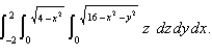# Calculus Early Transcendentals

Mathematics

## Quiz 12 : Multiple IntegralsStudy FlashcardsLooking for Calculus Homework Help?

## Quiz 12 :Multiple Integrals

Question TypeFind the center of mass of a homogeneous solid bounded by the paraboloid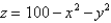and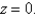Free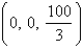Tags
Choose question tagUse cylindrical or spherical coordinates, whichever seems more appropriate, to evaluate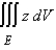where E lies above the paraboloid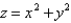and below the plane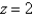.
Free
Multiple Choice

E

Tags
Choose question tagUse the transformation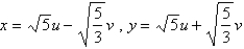to evaluate the integral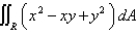, where R is the region bounded by the ellipse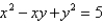.
Free
Multiple Choice

C

Tags
Choose question tagFind the mass of the solid S bounded by the paraboloid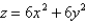and the plane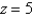if S has constant density 3.
Multiple Choice
Tags
Choose question tagFind the Jacobian of the transformation.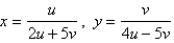Tags
Choose question tagUse cylindrical coordinates to evaluate the triple integral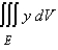where E is the solid that lies between the cylinders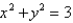and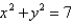above the xy-plane and below the plane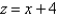.
Multiple Choice
Tags
Choose question tagUse a triple integral to find the volume of the solid bounded by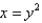and the planes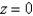and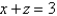.
Multiple Choice
Tags
Choose question tagFind the Jacobian of the transformation.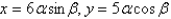Multiple Choice
Tags
Choose question tagUse the given transformation to evaluate the integral.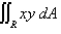, where R is the region in the first quadrant bounded by the lines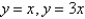and the hyperbolas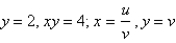.
Multiple Choice
Tags
Choose question tagIdentify the surface with equation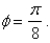Tags
Choose question tagIdentify the surface with equation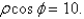Tags
Choose question tagUse cylindrical coordinates to evaluate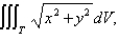where T is the solid bounded by the cylinder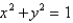and the planesand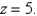Multiple Choice
Tags
Choose question tagFind the mass of a solid hemisphere of radius 5 if the mass density at any point on the solid is directly proportional to its distance from the base of the solid.
Multiple Choice
Tags
Choose question tagEvaluate the integral by making an appropriate change of variables. Round your answer to two decimal places.R is the parallelogram bounded by the lines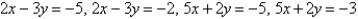.
Tags
Choose question tagCalculate the iterated integral.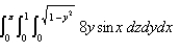Multiple Choice
Tags
Choose question tagUse spherical coordinates to find the volume of the solid that lies within the sphere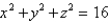above the xy-plane and below the cone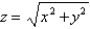. Round the answer to two decimal places.
Tags
Choose question tagUse the given transformation to evaluate the integral.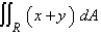, where R is the square with vertices (0, 0), (4, 6), (6,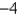), (10, 2) and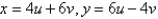Multiple Choice
Tags
Choose question tagUse spherical coordinates to find the moment of inertia of the solid homogeneous hemisphere of radius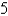and density 1 about a diameter of its base.
Multiple Choice
Tags
Choose question tagUse spherical coordinates.Evaluate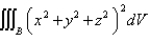, where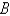is the ball with center the origin and radius.
Multiple ChoiceUse cylindrical coordinates to evaluate